IF YOU CAN SEE THIS go to /sfa/Nuprl/Shared/Xindentation_hack_doc.html

### Void

Void is an empty type, i.e., it has no members.

There are also other ways to express the empty type by means of other Nuprl primitives, such as,

Thm* Void =ext {x: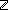x<x }

Thm* Void =ext rec(A.A)

Thm* Void =ext (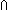x:. {x:}) since these singleton types are disjoint.

And of course, Thm* Void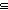A.

Here are a few examples of how Void relates to other type constructors.

Thm* Void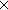A =ext Void

Thm* Void List =ext {nil:Void List}

Thm* Void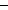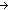A =ext {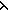x.x:VoidA}

The last theorem above states that VoidA has only the one member (x.x). This is a degenerate function in that it cannot be applied to anything in Void. Indeed, the body of the function expression is irrelevant since it will never be evaluated as part of application to any argument of type Void.

Thm* (x.whatever) = (x.x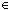VoidA

So, although VoidA has only one member, every (closed) lambda expression denotes that member. See Functions.

Similarly, the intersection over the empty domain is a trivial one-element type whose single member is denoted by every expression that can denote at all:

Thm* whatever1 = whatever2Whatever(given Void)

Thm* whatever1 = whatever2(x:Void. Whatever(x))

Sometimes this last type is denoted "Top" since:

Thm* ATop

where Def Top == Void(given Void).

See Polymorphism.

(Aug 2001 - sfa )